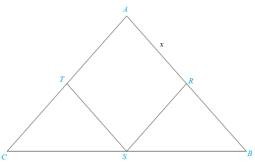Chapter 5.3, Problem 43EElementary Geometry For College St...

7th Edition
Alexander + 2 others
ISBN: 9781337614085

Solutions

Chapter
SectionElementary Geometry For College St...

7th Edition
Alexander + 2 others
ISBN: 9781337614085
Textbook Problem

The vertices of rhombus ARST lie on Δ A B C as shown. Where A B = c and A C = b , show that R S = b c b + c (Let A R = x .)To determine

To show:

The side RS=bcb+c by using the given information.

Explanation

Definition:

AA:

If two angles of one triangle are congruent to two angles of another triangle, then the triangles are similar.

CSSTP:

Corresponding sides of similar triangles are proportional.

Description:

Given that the vertices of rhombus ARST lie on ΔABC, where AB=c, AR=x and AC=b.

The given figure is shown below.

Figure

From the given figure, it is observed that vertices of rhombus ARST lie on ΔABC.

Also it is observed that RBS is a triangle.

It is known that the vertices of rhombus are the same and the opposite sides are parallel.

So, RS = x and RS¯AC¯.

If two parallel lines are cut by a transversal corresponding angles are congruent.

That is. RA.

Now consider two triangles ABC and RBS.

Here, both triangles have the common point B.

Hence, by identity BB.

The above mentioned AA definition, ΔABCΔRBS since two angles of one triangle are congruent to two angles of another triangle.

From the definition of CSSTP, corresponding sides of similar triangles are proportional.

That is, BRBA=BSBC=RSAC.

Consider first and last proportions to find the values of x

Still sussing out bartleby?

Check out a sample textbook solution.

See a sample solution

The Solution to Your Study Problems

Bartleby provides explanations to thousands of textbook problems written by our experts, many with advanced degrees!

Get Started

Evaluate the integral. 12s2+1s2ds

Calculus (MindTap Course List)

Use continuity to evaluate the limit. limxsin(x+sinx)

Single Variable Calculus: Early Transcendentals, Volume I

In Exercises 1728, use the logarithm identities to obtain the missing quantity.

Finite Mathematics and Applied Calculus (MindTap Course List)

The appropriate option for the value of (123).

Study Guide for Stewart's Single Variable Calculus: Early Transcendentals, 8th